# Week 8: Numerically Solving ODEs

I will do some things slightly differently, albeit equivallently, to what the older notes do. I'll show what I would change in the lecture video.

### Forward Euler

First, let's try to solve the initial value problem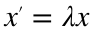and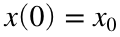with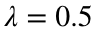and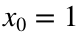. We know that the true solution is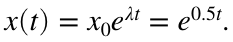We can plot this true solution with the code
lambda = 0.5;
x0 = 1;
x_true = @(t)(x0*exp(lambda * t));
tplot = 0:0.001:4;
plot(tplot, x_true(tplot), 'k')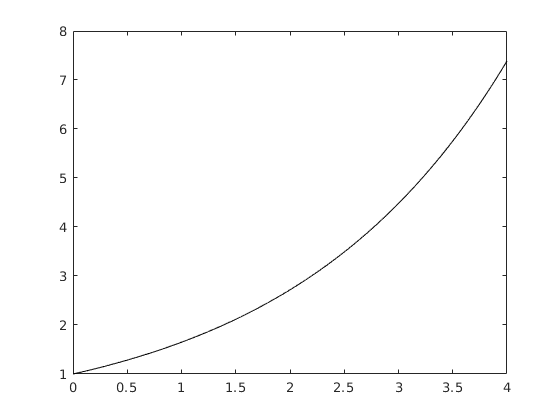If we want to solve this numerically, the first thing we have to do is choose the t-values that we want to approximate x at. Since we always choose evenly spaced t's that start at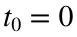, this means we have to choose a spacing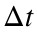and a final time T. For no particular reason, let's choose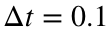and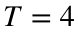.
dt = 0.1;
T = 4;
t = 0:dt:T;
We want to find an approximation of x at each of these t-values. The most convenient way to store these approximations in MATLAB is to make a vector of x's that is the same size as the vector t.
n = length(t);
x = zeros(1, n);
The first entry of x will contain the approximation at the first t, the second entry of x will contain the approximation at the second t, etc. In particular, we already know that the first entry of x should just be the initial condition.
x(1) = x0;
The meat of the algorithm comes when we fill in the rest of the vector x. We know that we need to use the formula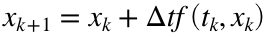. Since f is just the right hand side of our differential equation, we know that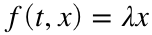, so the forward Euler equation is just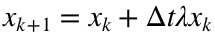. To use this formula over and over again, we should put it in a loop, so the code will look like
for k =
x(k+1) = x(k) + dt*lambda*x(k);
end
The only question is what to use for the bounds of k. Remember that we want to fill in all n entries of the vector x, one after the other. The first entry is already done, so the first one we have to do in the loop is x(2), which means k should start at 1. The last entry we have to do in the loop is x(n), which means k should end at n-1. This means that our final code should look like this:
for k = 1:n-1
x(k+1) = x(k) + dt*lambda*x(k);
end
We have now filled in the entire vector x and we can plot it to see how well we did.
tplot = 0:0.001:T;
plot(tplot, x_true(tplot), 'k', t, x, 'r')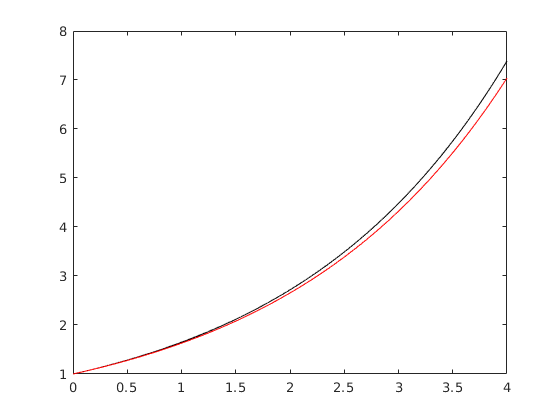This approximation doesn't look too bad. We can quantify just how good or bad it is by calculating the error. If we want to know the local accuracy, we can check the error at our first approximation (which is actually the second x-value, since the first one is exact).
err_local = abs(x(2) - x_true(t(2)))
err_local = 0.0013
If we want to know the global accuracy, we can check the error at our last approximation.
err_global = abs(x(end) - x_true(t(end)))
err_global = 0.3491
Another useful measure of accuracy is the maximum error. That is, we find the largest error out of all of our approximations. It turns out (and we will verify this for our examples) that the maximum error may or may not be the same value as the global error, but it does have the same order. (That is, it behaves the same way when we reduce.)
err_max = max(abs(x - x_true(t)))
err_max = 0.3491
We can confirm the local and global orders of accuracy by reducingand checking how these errors change. For instance, if we reduceby a factor of ten then we get
dt = 0.01;
T = 4;
t = 0:dt:T;
n = length(t);
x = zeros(1, n);
x(1) = x0;
for k = 1:n-1
x(k+1) = x(k) + dt*lambda*x(k);
end
err_local = abs(x(2) - x_true(t(2)))
err_local = 1.2521e-05
err_global = abs(x(end) - x_true(t(end)))
err_global = 0.0367
err_max = max(abs(x - x_true(t)))
err_max = 0.0367
Notice that the local error went down by a factor of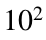, while the global and maximum errors only went down by a factor of 10. This confirms that forward Euler has second order local accuracy and first order global accuracy.
We can also check the stability of forward Euler by solving this initial value problem over a much longer time. Since the true solution is unstable, we expect that our forward Euler approximation will also be unstable, regardless of how big we make.
dt = 10;
T = 200;
t = 0:dt:T;
n = length(t);
x = zeros(1, n);
x(1) = x0;
for k = 1:n-1
x(k+1) = x(k) + dt*lambda*x(k);
end
plot(t, x, 'r')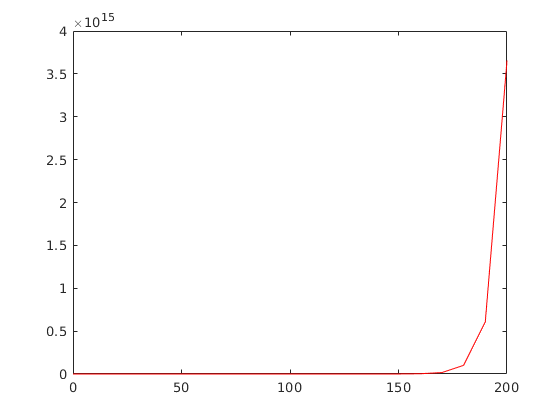As you can see, the approximation does go to infinity, even with a very large. (We didn't calculate the errors here, but it's worth noting that the global error is enormous. In general, if the true solution goes to infinity then there is no hope of getting a particularly small error. The best we can hope for is that we get the general shape of the solution correct.)
Now let's try the same thing, but with a different value of λ. In particular, let's choose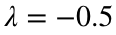. The code is the same, except that we change the value of λ. We find
lambda = -0.5;
x0 = 1;
x_true = @(t)(x0*exp(lambda * t));
dt = 0.1;
T = 4;
t = 0:dt:T;
n = length(t);
x = zeros(1, n);
x(1) = x0;
for k = 1:n-1
x(k+1) = x(k) + dt*lambda*x(k);
end
tplot = 0:0.001:T;
plot(tplot, x_true(tplot), 'k', t, x, 'r')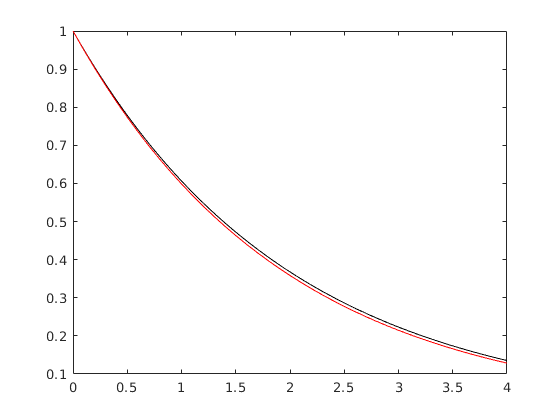err_local = abs(x(2) - x_true(t(2)))
err_local = 0.0012
err_global = abs(x(end) - x_true(t(end)))
err_global = 0.0068
err_max = max(abs(x - x_true(t)))
err_max = 0.0094
As before, we can confirm the local and global accuracy by seeing how these errors change as we decrease. We get
dt = 0.01;
T = 4;
t = 0:dt:T;
n = length(t);
x = zeros(1, n);
x(1) = x0;
for k = 1:n-1
x(k+1) = x(k) + dt*lambda*x(k);
end
tplot = 0:0.001:T;
plot(tplot, x_true(tplot), 'k', t, x, 'r')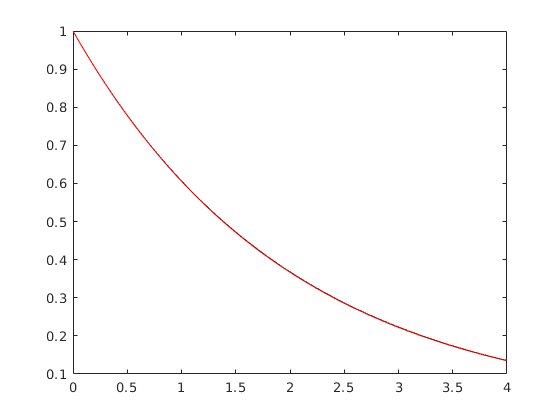err_local = abs(x(2) - x_true(t(2)))
err_local = 1.2479e-05
err_global = abs(x(end) - x_true(t(end)))
err_global = 6.7724e-04
err_max = max(abs(x - x_true(t)))
err_max = 9.2162e-04
As you can see, the local error dropped by a factor of, while the global and maximum errors dropped by a factor of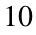. This confirms once again that forward Euler has second order local accuracy and first order global accuracy.
To test the stability properties, we should solve the same initial value problem over a much longer time period. The true solution is stable, so we would like our approximation to go to zero, but we already know from our stability analysis that ifis too large then the approximation will go to infinity. We get
dt = 10;
T = 200;
t = 0:dt:T;
n = length(t);
x = zeros(1, n);
x(1) = x0;
for k = 1:n-1
x(k+1) = x(k) + dt*lambda*x(k);
end
plot(t, x, 'r')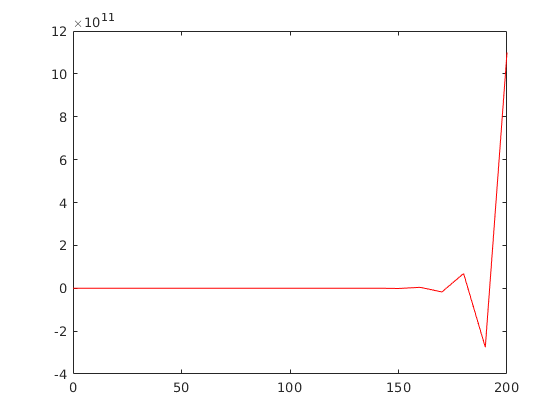This confirms our stability analysis.

### Backward Euler

Now we will try to solve the same problems with the backward Euler method. To start, let's use. All of the setup code is exactly the same, so we still start with
lambda = 0.5;
x0 = 1;
x_true = @(t)(x0*exp(lambda * t));
dt = 0.1;
T = 4;
t = 0:dt:T;
n = length(t);
x = zeros(1, n);
x(1) = x0;
The only difference comes in our for loop. Instead of the forward Euler equation, we need to use the formula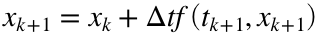. The function f is just the right hand side of our differential equation, soand our backward Euler equation becomes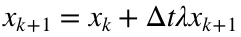.
This is not actually solved for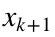yet, but it is easy to solve by hand. We get the formulaWe can therefore rewrite our for loop as
for k = 1:n-1
x(k+1) = x(k) / (1 - dt*lambda);
end
We can now check the quality of our approximation by graphing it
tplot = 0:0.001:T;
plot(tplot, x_true(tplot), 'k', t, x, 'r')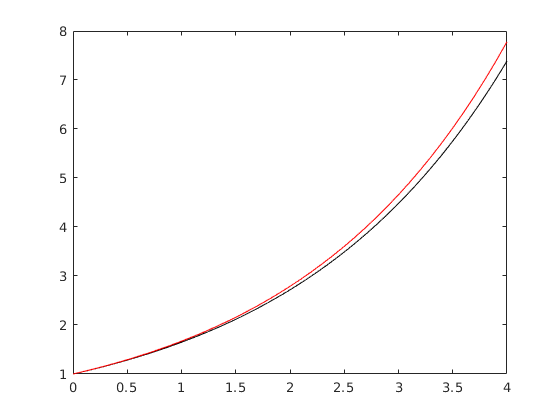or by calculating the various errors.
err_local = abs(x(2) - x_true(t(2)))
err_local = 0.0014
err_global = abs(x(end) - x_true(t(end)))
err_global = 0.3923
err_max = max(abs(x - x_true(t)))
err_max = 0.3923
To confirm the local and global accuracy, we can check what happens when we shrink. For example, if we shrinkby a factor of 10 then we get
dt = 0.01;
T = 4;
t = 0:dt:T;
n = length(t);
x = zeros(1, n);
x(1) = x0;
for k = 1:n-1
x(k+1) = x(k) / (1 - dt*lambda);
end
err_local = abs(x(2) - x_true(t(2)))
err_local = 1.2605e-05
err_global = abs(x(end) - x_true(t(end)))
err_global = 0.0372
err_max = max(abs(x - x_true(t)))
err_max = 0.0372
Notice that, once again, the local error shrank by a factor ofand the global and maximum error shrank by a factor of 10. This confirms that backward Euler has second order local accuracy and first order global accuracy.
We can also check the stability of backward Euler by solving this initial value problem over a much longer time. Since the true solution is unstable, we expect that our backward Euler approximation will become stable if we makesufficiently large. That is, the true solution goes to infinity, but if we maketoo big then our approximation will go to zero instead.
dt = 10;
T = 200;
t = 0:dt:T;
n = length(t);
x = zeros(1, n);
x(1) = x0;
for k = 1:n-1
x(k+1) = x(k) / (1 - dt*lambda);
end
plot(t, x, 'r')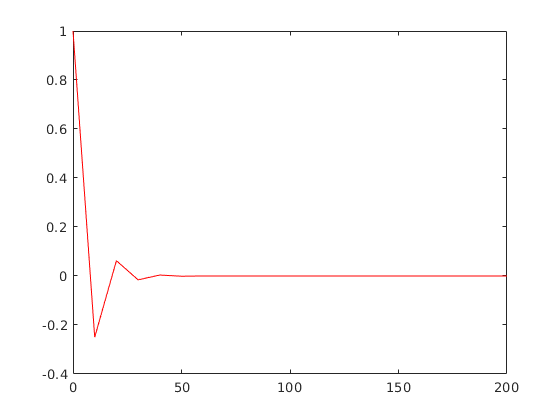This confirms our stability analysis.
If we switch to, we get similar results. For example, if we choosethen we get
lambda = -0.5;
x0 = 1;
x_true = @(t)(x0*exp(lambda * t));
dt = 0.1;
T = 4;
t = 0:dt:T;
n = length(t);
x = zeros(1, n);
x(1) = x0;
for k = 1:n-1
x(k+1) = x(k) / (1 - dt*lambda);
end
err_local = abs(x(2) - x_true(t(2)))
err_local =
0.001151527880238
err_global = abs(x(end) - x_true(t(end)))
err_global =
0.006710399063665
err_max = max(abs(x - x_true(t)))
err_max =
0.009010041701558
If we then shrinkby a factor of ten, we get
dt = 0.01;
T = 4;
t = 0:dt:T;
n = length(t);
x = zeros(1, n);
x(1) = x0;
for k = 1:n-1
x(k+1) = x(k) / (1 - dt*lambda);
end
err_local = abs(x(2) - x_true(t(2)))
err_local =
1.239642920836115e-05
err_global = abs(x(end) - x_true(t(end)))
err_global =
6.761125217469854e-04
err_max = max(abs(x - x_true(t)))
err_max =
9.177873408658388e-04
Notice that the local error decreased by a factor of, while the global and maximum error only decreased by a factor of 10. This confirms our accuracy analysis.
To test the stability properties, we should solve the same initial value problem over a much longer time period. The true solution is stable, so we would like our approximation to go to zero as well. We already know from our stability analysis that the backward Euler approximation should remain stable no matter how largeis. For instance,
dt = 10;
T = 200;
t = 0:dt:T;
n = length(t);
x = zeros(1, n);
x(1) = x0;
for k = 1:n-1
x(k+1) = x(k) / (1 - dt*lambda);
end
tplot = 0:0.001:T;
plot(tplot, x_true(tplot), 'k', t, x, 'r')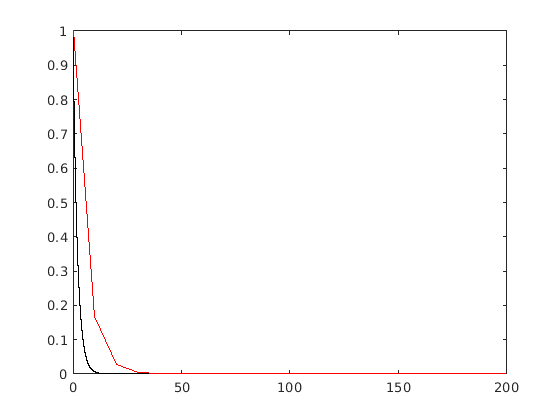Notice that our approximation is quite bad for the first several time steps, because we chose a very large, but we still got the long term behavior correct. This confirms our stability analysis for the backward Euler method.

## Builtin Solvers

MATLAB has several builtin functions for solving differential equations. The most popular is called ode45, but there are a few others. The syntax for all of these solvers is exactly the same, so we will focus on ode45. (As a side note, ODE stands for ordinary differential equation. The word ordinary means that we are solving for a function of only one variable. That is, x is just a function of t, not a function of t and y. We will only look at ordinary differential equations in this class, so you can just translate ODE to "differential equation".)
The syntax for ode45 is
[vector of t-values, vector of x-values] = ode45(function, time span, initial condition)
The outputs are essentially the same as the vectors t and x that we made in our forward and backward Euler code. The "function" input should be a MATLAB function for the right hand side of the differential equation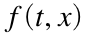. The time span is a vector whose first entry is the initial time (always zero in this class) and whose last entry is the final time T. The initial condition is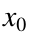. The one special rule is that the function must always be a function of two variables, and the first variable should always be time. This is easy to forget because a lot of our examples don't actually include t in the right hand side, but you have to include the variable t anyways.
For example, to solve the initial value problem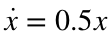,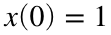up to time, we would use the code
f = @(t, x)(0.5*x);
tspan = [0 4];
x0 = 1;
[t, x] = ode45(f, tspan, x0)
t = 41×1
0 0.100000000000000 0.200000000000000 0.300000000000000 0.400000000000000 0.500000000000000 0.600000000000000 0.700000000000000 0.800000000000000 0.900000000000000
x = 41×1
1.000000000000000 1.051271196708333 1.105171054000000 1.161834301375000 1.221402773333333 1.284025555185010 1.349858990363323 1.419067637853221 1.491824734706358 1.568312374133845
Notice that t and x are column vectors, whereas our code for forward and backward Euler produced row vectors.
It is important to note that we did not actually specify ain this code. The ode45 function chooses its own spacing for the vector t. In this example, it looks like MATLAB chose, but it is possible for ode45 to choose any, and even to choose different spacings between different points. In general, it tries to makesmall enough that the global error is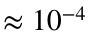.
You can force ode45 to find x at a specified vector of times by giving a whole vector for tspan instead of just two numbers. For example, we could have written
f = @(t, x)(0.5*x);
dt = 0.5;
tspan = 0:dt:4;
x0 = 1;
[t, x] = ode45(f, tspan, x0)
t = 9×1
0 0.500000000000000 1.000000000000000 1.500000000000000 2.000000000000000 2.500000000000000 3.000000000000000 3.500000000000000 4.000000000000000
x = 9×1
1.000000000000000 1.284025555185010 1.648721514438696 2.117000202370759 2.718281997301530 3.490343550734517 4.481690011262423 5.754603538388132 7.389057016853601
It looks like this forced ode45 to use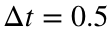, but that's not actually what happened. MATLAB chose its own spacing (in particular, it chose the same t-values as we got in the last example) and then used an interpolation method (like the ones we discussed a few weeks ago) to approximate x at the times you asked for. This means that the error will not actually go down if you decrease dt in this code.
I will often ask you to specify t-values like this on the homework, because it will ensure that MATLAB and python users get similar answers.
We will talk about how to implement the method used by ode45 next week, but for now you should just assume that this value is extremely close to the true solution.